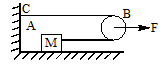A block ‘A’ of mass m is tied to a fixed point C on a horizontal table through a string passing            round a massless smooth pulley B. A force F is applied by the experimenter to the pulley. Show            that if the pulley is displaced by the distance x, the block will be displaced by 2x. The            acceleration of the block and the pulley.

# A block ‘A’ of mass m is tied to a fixed point C on a horizontal table through a string passing            round a massless smooth pulley B. A force F is applied by the experimenter to the pulley. Show            that if the pulley is displaced by the distance x, the block will be displaced by 2x. The            acceleration of the block and the pulley.1. A

$\frac{\text{F}}{\text{2m}}\text{,\hspace{0.17em}}\frac{\text{F}}{\text{4m}}$

2. B

$\frac{\text{F}}{\text{m}}\text{,\hspace{0.17em}}\frac{\text{F}}{\text{4m}}$

3. C

$\frac{\text{F}}{\text{2m}}\text{,\hspace{0.17em}}\frac{\text{F}}{\text{m}}$

4. D

$\frac{\text{2F}}{\text{m}}\text{,\hspace{0.17em}}\frac{\text{F}}{\text{m}}$

Register to Get Free Mock Test and Study Material

+91

Verify OTP Code (required)